# structuralDamping

Specify damping parameters for transient or frequency response structural model

## Syntax

``structuralDamping(structuralmodel,'Alpha',a,'Beta',b)``
``structuralDamping(structuralmodel,'Zeta',z)``
``damping = structuralDamping(___)``

## Description

example

````structuralDamping(structuralmodel,'Alpha',a,'Beta',b)` specifies proportional (Rayleigh) damping parameters `a` and `b` for a `structuralmodel` object.For a frequency response model with damping, the results are complex. Use the `abs` and `angle` functions to obtain real-valued magnitude and phase, respectively.```

example

````structuralDamping(structuralmodel,'Zeta',z)` specifies the modal damping ratio. Use this parameter when you solve a transient or frequency response model using the results of modal analysis.```
````damping = structuralDamping(___)` returns the damping parameters object, using any of the previous input syntaxes.```

## Examples

collapse all

Specify proportional (Rayleigh) damping parameters for a beam.

Create a transient structural model.

` structuralModel = createpde('structural','transient-solid');`

Import and plot the geometry.

``` gm = importGeometry(structuralModel,'SquareBeam.stl'); pdegplot(structuralModel,'FaceAlpha',0.5)```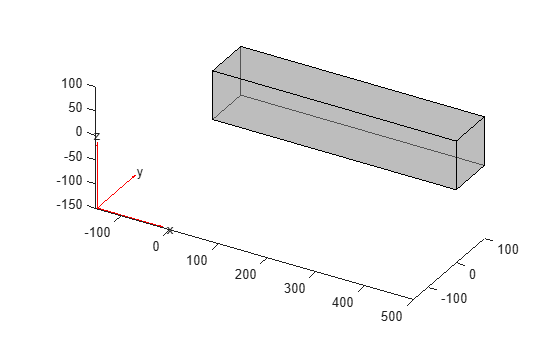Specify Young's modulus, Poisson's ratio, and the mass density.

```structuralProperties(structuralModel,'YoungsModulus',210E9,... 'PoissonsRatio',0.3,... 'MassDensity',7800);```

Specify the Rayleigh damping parameters.

`structuralDamping(structuralModel,'Alpha',10,'Beta',2)`
```ans = StructuralDampingAssignment with properties: RegionType: 'Cell' RegionID: 1 DampingModel: "proportional" Alpha: 10 Beta: 2 Zeta: [] ```

Solve a frequency response problem with damping. The resulting displacement values are complex. To obtain the magnitude and phase of displacement, use the `abs` and `angle` functions, respectively. To speed up computations, solve the model using the results of modal analysis.

Modal Analysis

Create a modal analysis model for a 3-D problem.

`modelM = createpde('structural','modal-solid');`

Create the geometry and include it in the model.

```gm = multicuboid(10,10,0.025); modelM.Geometry = gm;```

Generate a mesh.

`msh = generateMesh(modelM);`

Specify Young's modulus, Poisson's ratio, and the mass density of the material.

```structuralProperties(modelM,'YoungsModulus',2E11, ... 'PoissonsRatio',0.3, ... 'MassDensity',8000);```

Identify faces for applying boundary constraints and loads by plotting the geometry with the face and edge labels.

`pdegplot(gm,'FaceLabels','on','FaceAlpha',0.5)`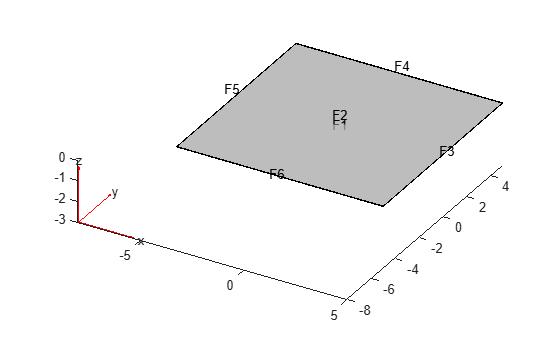```figure pdegplot(gm,'EdgeLabels','on','FaceAlpha',0.5)```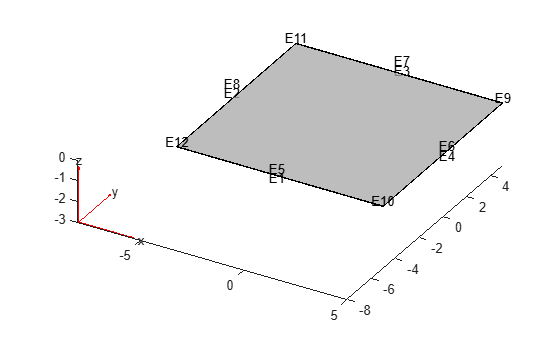Specify constraints on the sides of the plate (faces 3, 4, 5, and 6) to prevent rigid body motions.

`structuralBC(modelM,'Face',[3,4,5,6],'Constraint','fixed');`

Solve the problem for the frequency range from `-Inf` to` 12*pi`.

`Rm = solve(modelM,'FrequencyRange',[-Inf,12*pi]);`

Frequency Response Analysis

Create a frequency response analysis model for a 3-D problem.

`modelFR = createpde('structural','frequency-solid');`

Use the same geometry and mesh as you used for the modal analysis.

```modelFR.Geometry = gm; modelFR.Mesh = msh;```

Specify the same values for Young's modulus, Poisson's ratio, and the mass density of the material.

```structuralProperties(modelFR,'YoungsModulus',2E11, ... 'PoissonsRatio',0.3, ... 'MassDensity',8000);```

Specify the same constraints on the sides of the plate to prevent rigid body modes.

`structuralBC(modelFR,'Face',[3,4,5,6],'Constraint','fixed');`

Specify the pressure loading on top of the plate (face 2) to model an ideal impulse excitation. In the frequency domain, this pressure pulse is uniformly distributed across all frequencies.

`structuralBoundaryLoad(modelFR,'Face',2,'Pressure',1E2);`

First, solve the model without damping.

```flist = [0,1,1.5,linspace(2,3,100),3.5,4,5,6]*2*pi; RfrModalU = solve(modelFR,flist,'ModalResults',Rm);```

Now, solve the model with damping equal to 2% of critical damping for all modes.

```structuralDamping(modelFR,'Zeta',0.02); RfrModalAll = solve(modelFR,flist,'ModalResults',Rm);```

Solve the same model with frequency-dependent damping. In this example, use the solution frequencies from `flist` and damping values between 1% and 10% of critical damping.

```omega = flist; zeta = linspace(0.01,0.1,length(omega)); zetaW = @(omegaMode) interp1(omega,zeta,omegaMode); structuralDamping(modelFR,'Zeta',zetaW); RfrModalFD = solve(modelFR,flist,'ModalResults',Rm);```

Interpolate the displacement at the center of the top surface of the plate for all three cases.

```iDispU = interpolateDisplacement(RfrModalU,[0;0;0.025]); iDispAll = interpolateDisplacement(RfrModalAll,[0;0;0.025]); iDispFD = interpolateDisplacement(RfrModalFD,[0;0;0.025]);```

Plot the magnitude of the displacement. Zoom in on the frequencies around the first mode.

```figure hold on plot(RfrModalU.SolutionFrequencies,abs(iDispU.Magnitude)); plot(RfrModalAll.SolutionFrequencies,abs(iDispAll.Magnitude)); plot(RfrModalFD.SolutionFrequencies,abs(iDispFD.Magnitude)); title('Magnitude') xlim([10 25]) ylim([0 0.5])```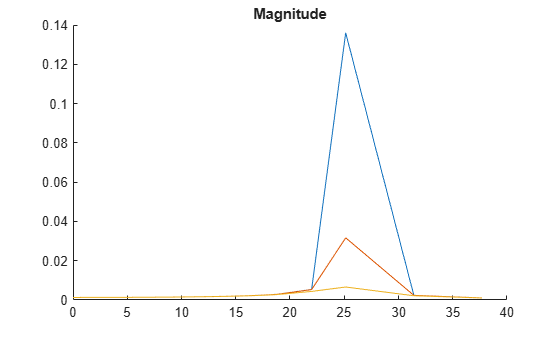Plot the phase of the displacement.

```figure hold on plot(RfrModalU.SolutionFrequencies,angle(iDispU.Magnitude)); plot(RfrModalAll.SolutionFrequencies,angle(iDispAll.Magnitude)); plot(RfrModalFD.SolutionFrequencies,angle(iDispFD.Magnitude)); title('Phase')```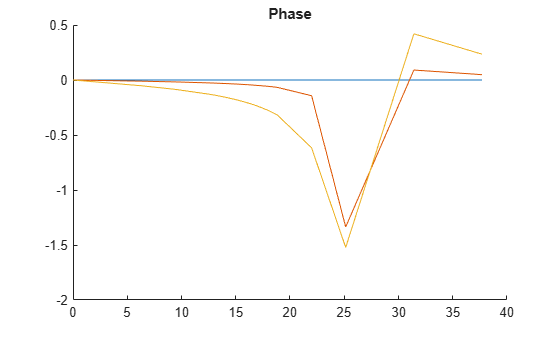## Input Arguments

collapse all

Transient or frequency response structural model, specified as a `StructuralModel` object. The model contains the geometry, mesh, structural properties of the material, body loads, boundary loads, boundary conditions, and initial conditions.

Example: ```structuralmodel = createpde('structural','transient-solid')```

Mass proportional damping, specified as a nonnegative number.

Data Types: `double`

Stiffness proportional damping, specified as a nonnegative number.

Data Types: `double`

Modal damping ratio, specified as a nonnegative number or a function handle. Use a function handle when each mode has its own damping ratio. The function must accept a vector of natural frequencies as an input argument and return a vector of corresponding damping ratios. It must cover the full frequency range for all modes used for modal solution. For details, see Modal Damping Depending on Frequency.

Data Types: `double` | `function_handle`

## Output Arguments

collapse all

Handle to damping parameters, returned as a `StructuralDampingAssignment` object. See StructuralDampingAssignment Properties.

collapse all

### Modal Damping Depending on Frequency

To use the individual value of modal damping for each mode, specify `z` as a function of frequency.

`function z = dampingFcn(omega)`

Typically, the damping ratio function is a linear interpolation of frequency versus the modal damping parameter:

```structuralDamping(modelD,'Zeta',@(omegaMode) ... interp1(omega,zeta,omegaMode))```

Here, `omega` is a vector of frequencies, and `zeta` is a vector of corresponding damping ratio values.

## Version History

Introduced in R2018a